# Empirical distribution

(diff) ← Older revision | Latest revision (diff) | Newer revision → (diff)

sample distribution

A probability distribution determined from a sample for the estimation of a true distribution. Suppose that resultsof observations are independent identically-distributed random variables with distribution functionand let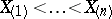be the corresponding order statistics. The empirical distribution corresponding tois defined as the discrete distribution that assigns to every value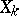the probability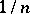. The empirical distribution functionis the step-function with steps of multiples ofat the points defined by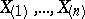:For fixed values ofthe function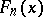has all the properties of an ordinary distribution function. For every fixed realthe functionis a random variable as a function of. Thus, the empirical distribution corresponding to a sampleis given by the family of random variablesdepending on the real parameter. Here for a fixed,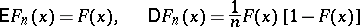andIn accordance with the law of large numbers,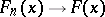with probability one asfor every. This means thatis an unbiased and consistent estimator of the distribution function. The empirical distribution function converges, uniformly in, with probability 1 to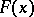as, i.e., ifthen(the Glivenko–Cantelli theorem).

The quantityis a measure of the proximity ofto. A.N. Kolmogorov found (in 1933) its limit distribution: For a continuous function,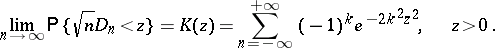Ifis not known, then to verify the hypothesis that it is a given continuous function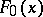one uses tests based on statistics of type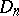(see Kolmogorov test; Kolmogorov–Smirnov test; Non-parametric methods in statistics).

Moments and any other characteristics of an empirical distribution are called sample or empirical; for example,is the sample mean,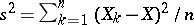is the sample variance, and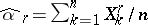is the sample moment of order.

Sample characteristics serve as statistical estimators of the corresponding characteristics of the original distribution.

How to Cite This Entry:
Empirical distribution. Encyclopedia of Mathematics. URL: http://encyclopediaofmath.org/index.php?title=Empirical_distribution&oldid=11280
This article was adapted from an original article by A.V. Prokhorov (originator), which appeared in Encyclopedia of Mathematics - ISBN 1402006098. See original article